Courses

# Ex 4.4 NCERT Solutions - Linear Equations in Two Variables Class 9 Notes | EduRev

## Class 9 Mathematics by Full Circle

Created by: Full Circle

## Class 9 : Ex 4.4 NCERT Solutions - Linear Equations in Two Variables Class 9 Notes | EduRev

The document Ex 4.4 NCERT Solutions - Linear Equations in Two Variables Class 9 Notes | EduRev is a part of the Class 9 Course Class 9 Mathematics by Full Circle.
All you need of Class 9 at this link: Class 9

Question 1. Give the geometric representations of y = 3 as an equation
(i) in one variable
(ii) in two variables
Solution: (i) y = 3 [An equation in one variable]
∵ y = 3 is an equation in one variable, i.e. y only.
∴ It has a unique solution y = 3 as shown on the number line shown here.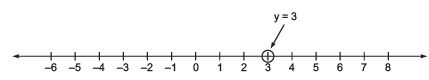The unique solution is a point.

(ii) y = 3 [An equation in two variables]
We can write y = 3 as
0.x + y = 3
Now, when x = 1, y = 3
when x = 2, y = 3
when x = 3, y = 3
We get the following table:

 x 1 2 3 y 3 3 3

Plotting the ordered pairs (1, 3), (2, 3) and (3, 3) on a graph paper, we get a line AB as solution of 0 . x + y = 3, i.e. y = 3.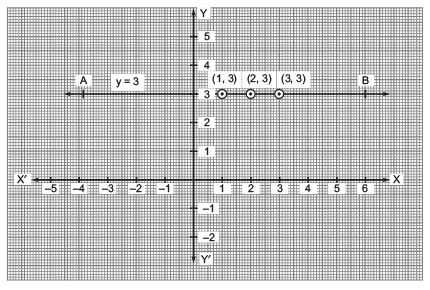Question 2. Give the geometric representations of 2x + 9 = 0 as an equation (i) in one variable (ii) in two variables
Solution: (i) 2x + 9 = 0 [An equation in one variable]
We have: 2x + 9 = 0
⇒ 2x = –9
⇒ x = (-9/2)
which is a linear equation in one variable ‘x’ only. Its solution is the point (-9/2) on the number line as shown below.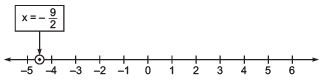(ii) 2x + 9 = 0 [An equation in two variables]
We can write 2x + 9 = 0 as 2x + 0y + 9 = 0 or 2x = –9 + 0y
or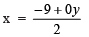∴ When y = 1,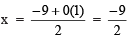When y = 2,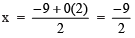When y = 3,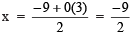Thus, we get the following table:

 x -9/2 -9/2 -9/2 y 1 2 3

Now, plotting the ordered pairs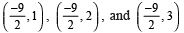on a graph paper and joining them, we get a line PQ as solution of 2x + 9 = 0.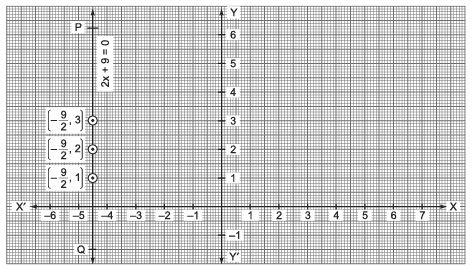132 docs

,

,

,

,

,

,

,

,

,

,

,

,

,

,

,

,

,

,

,

,

,

;